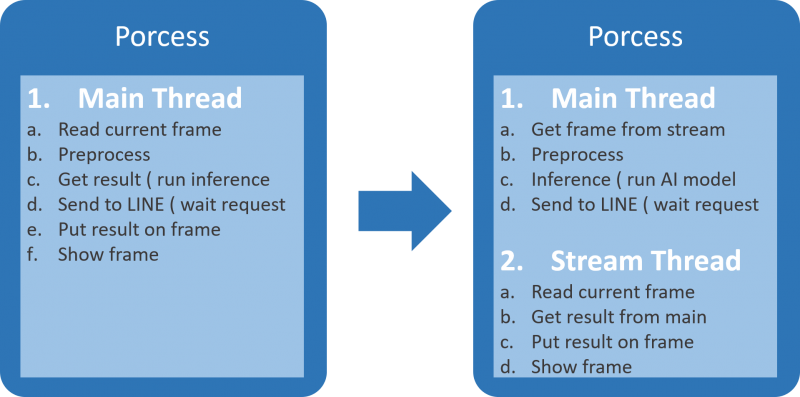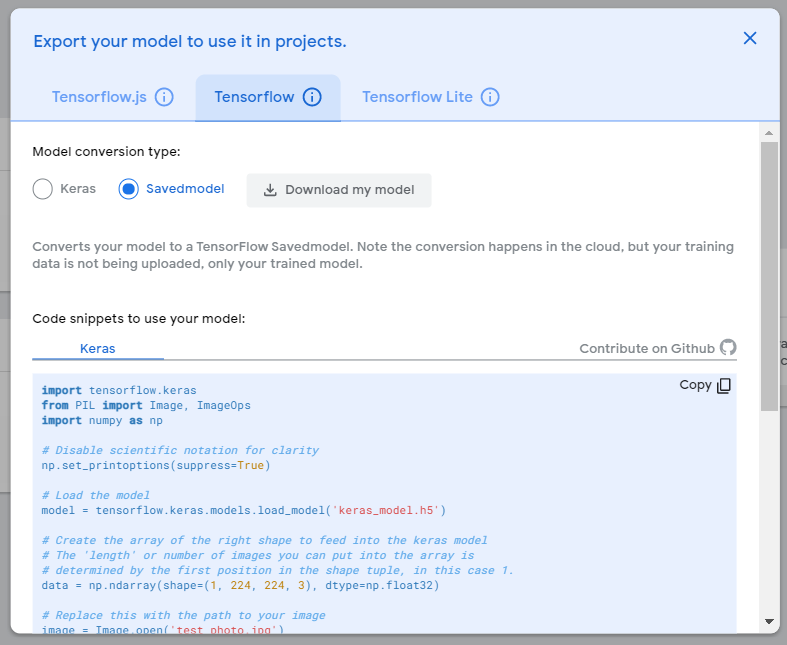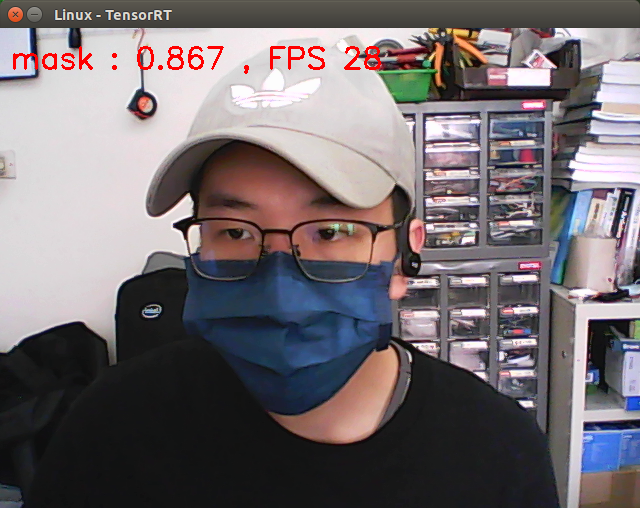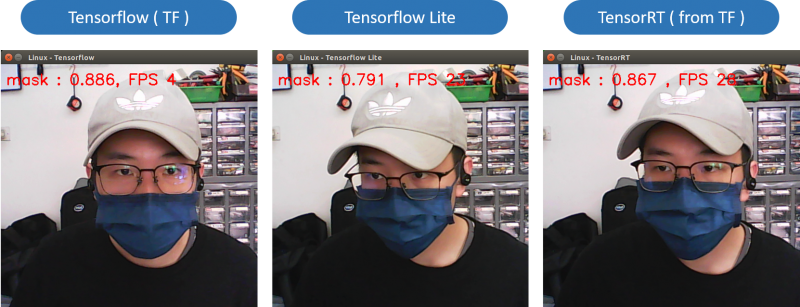# NVIDIA Jetson Nano應用-多執行緒平行處理，以專案「你帶口罩了嗎?」為例

*本文由RS components 贊助發表，轉載自DesignSpark部落格原文連結

 作者/攝影 張嘉鈞 難度 ★★☆☆☆（普通） 材料表 NVIDIA Jestson Nano Developer Kit PC or Notebook RK-NVIDIA® Jetson Nano™ Developer Kit B01 套件

### 思路分析### 平行運算中的多執行緒

1. 每個CPU都只能運行一個Process，每個Process彼此之間是獨立的。

### 客製化的即時影像物件

``# 客製化的影像擷取程式class CustomVideoCapture():    # 初始化 預設的攝影機裝置為 0    def __init__(self, dev=0):        self.cap = cv2.VideoCapture(dev)        self.ret = ''        self.frame = []        self.win_title = 'Modified with set_title()'        self.info = ''        self.fps = 0        self.fps_time = 0        self.isStop = False        self.t = threading.Thread(target=self.video, name='stream')``

``# 可以透過這個函式 開啟 Thread     def start_stream(self):        self.t.start()        # 關閉 Thread 與 Camera    def stop_stream(self):        self.isStop = True        self.cap.release()        cv2.destroyAllWindows()    # 取得最近一次的幀    def get_current_frame(self):        return self.ret, self.frame    def get_fps(self):        return self.fps    # 設定顯示視窗的名稱    def set_title(self, txt):        self.win_title = txt``

``# Thread主要運行的函式    def video(self):        try:            while(not self.isStop):                self.fps_time = time.time()                self.ret, self.frame = self.cap.read()                if self.info is not '':                    cv2.putText(self.frame, self.info, (10,40), cv2.FONT_HERSHEY_SIMPLEX, 1, (0,0,255), 2)                                cv2.imshow(self.win_title, self.frame)                                if cv2.waitKey(1) == ord('q'):                    break                                self.fps = int(1/(time.time() - self.fps_time))            self.stop_stream()        except:            self.stop_stream()``

``# 用於資料前處理的程式def preprocess(frame, resize=(224, 224), norm=True):    '''    設定格式 ( 1, 224, 224, 3)、縮放大、正規化、放入資料並回傳正確格式的資料    '''    input_format = np.ndarray(shape=(1, 224, 224, 3), dtype=np.float32)    frame_resize = cv2.resize(frame, resize)    frame_norm =  ((frame_resize.astype(np.float32) / 127.0) - 1) if norm else frame_resize    input_format=frame_norm    return input_format``

``# 讀取 模型 與 標籤def load_model_folder(trg_dir) -> "'trg_dir' is the path include model file and labels file. return (model, label).":    model_type = [ 'trt','engine','h5']    label_type = [ 'txt']    for f in os.listdir(trg_dir):        extension = f.split('.')[-1]                if extension in model_type:            model_dir = os.path.join(trg_dir, f)        elif extension in label_type:            lable_dir = os.path.join(trg_dir, f)    return get_model(model_dir), get_label(lable_dir)``

``# 讀取模型def get_model(model_dir) -> "support keras and tensorrt model":        if model_dir.split('.')[-1] == 'h5':        print('Load Keras Model')        model = tf.keras.models.load_model(model_dir)    else:        print('Load TensorRT Engine')        model = load_engine(model_dir)            return model    # 讀取標籤def get_label(lable_dir) -> 'return dict of labels':    label = {}    with open(lable_dir) as f:            for line in f.readlines():            idx, name = line.strip().split(' ')            label[int(idx)]=name    return label# 讀取TensorRT模型def load_engine(engine_path):    if trt_found:        TRT_LOGGER = trt.Logger(trt.Logger.WARNING)        trt_runtime = trt.Runtime(TRT_LOGGER)                with open(engine_path, 'rb') as f:            engine_data = f.read()        engine = trt_runtime.deserialize_cuda_engine(engine_data)        return engine    else:        print("Can not load load_engine because there is no tensorrt module")        exit(1)``

``# 解析輸出資訊def parse_output(preds, label) -> 'return ( class id, class name, probobility) ':        preds = preds if len(preds.shape)==4 else preds    trg_id = np.argmax(preds)    trg_name = label[trg_id]    trg_prob = preds[trg_id]    return ( trg_id, trg_name, trg_prob)``

1.取得模型與標籤、開啟即時影像的線程：

``# 取得模型與標籤model, label = load_model_folder('keras_models')# 設定影像擷取vid = CustomVideoCapture()vid.set_title('{sys} - {framework}'.format(sys='Jetson Nano', framework='Tensorflow'))vid.start_stream()``

2.設定辨識的參數，主要用於控制幾秒辨識一次 ( t_delay )，與上次辨識結果不同才進行傳送 ( pre_id )：

``# 設定幾秒辨識一次，降低運行負擔t_check = 0t_delay = 2t_start = 0# 儲存上一次辨識的結果，如果改變才傳送，防止ifttt負擔太大pre_id = -1``

3.設定IFTTT的參數：

``# 設定「Line訊息」資訊event = 'jetsonnano_line'key = 'i3_S_gIAsOty30yvIg4vg'status = {    0:['是本人', '確定有做好防疫工作'],    1:['是本人', '注意，已成為防疫破口'],     2:['離開位置', ''],     3:['非本人', '注意您的財產']    }``

4.使用While不斷進行即時的辨識與LINE監控，這邊設定了如果大於預設的delay時間則進行辨識：

``# 開始即時辨識t_start = time.time()while(not vid.isStop):    # 計算時間如果大於預設延遲時間則進行辨識與發送    t_check = time.time() - t_start    if (t_check >= t_delay) or ( not vid.fps):        # 取得當前圖片        ret, frame = vid.get_current_frame()        # 如果沒有幀則重新執行        if not ret: continue``

5.進行推論以及取得辨識結果，最後設定顯示在即時影像上的資訊：

``# 進行處理與推論        data = preprocess(frame, resize=(224,224), norm=True)        prediction = model(data)                # 解析 辨識結果        trg_id, trg_class, trg_prob =parse_output(prediction, label)        # 設定顯示資訊        vid.info = '{} : {:.3f} , FPS {}'.format(trg_class, trg_prob, vid.get_fps())``

6.如果辨識結果與上次的不同，則回傳給LINE：

``        if pre_id != trg_id:                        ifttt.send_to_webhook(event,                                     key,                                     '環境變動',                                     status[trg_id],                                     status[trg_id] if status[trg_id] else '')            pre_id = trg_id        # 更新 time        t_start = time.time()``

``# 跳出 while 迴圈需要檢查多線程是否已經關閉time.sleep(1)print('-'*30)print(f'影像串流的線程是否已關閉 : {not vid.t.is_alive()}')print('離開程式')``

``#%%import cv2import threadingimport os, time, randomimport iftttimport numpy as npimport tensorflow as tfimport platform as pltfrom tools import CustomVideoCapture, preprocess, load_model_folder, parse_outputimport time# 取得模型與標籤model, label = load_model_folder('keras_models')# 設定影像擷取vid = CustomVideoCapture()vid.set_title('{sys} - {framework}'.format(sys='Jetson Nano', framework='Tensorflow'))vid.start_stream()# 設定幾秒辨識一次，降低運行負擔t_check = 0t_delay = 2t_start = 0# 儲存上一次辨識的結果，如果改變才傳送，防止ifttt負擔太大pre_id = -1# 設定「Line訊息」資訊event = 'jetsonnano_line'key = 'i3_S_gIAsOty30yvIg4vg'status = {    0:['是本人', '確定有做好防疫工作'],    1:['是本人', '注意，已成為防疫破口'],     2:['離開位置', ''],     3:['非本人', '注意您的財產']    }#%%                             # 開始即時辨識t_start = time.time()while(not vid.isStop):    # 計算時間如果大於預設延遲時間則進行辨識與發送    t_check = time.time() - t_start    if (t_check >= t_delay) or ( not vid.fps):        # 取得當前圖片        ret, frame = vid.get_current_frame()        # 如果沒有幀則重新執行        if not ret: continue        # 進行處理與推論        data = preprocess(frame, resize=(224,224), norm=True)        prediction = model(data)                # 解析 辨識結果        trg_id, trg_class, trg_prob =parse_output(prediction, label)        # 設定顯示資訊        vid.info = '{} : {:.3f} , FPS {}'.format(trg_class, trg_prob, vid.get_fps())        # 如果與上次辨識不同，則將辨識到的結果傳送至Line        if pre_id != trg_id:                        ifttt.send_to_webhook(event,                                     key,                                     '環境變動',                                     status[trg_id],                                     status[trg_id] if status[trg_id] else '')            pre_id = trg_id        # 更新 time        t_start = time.time()# 跳出 while 迴圈需要檢查多線程是否已經關閉time.sleep(1)print('-'*30)print(f'影像串流的線程是否已關閉 : {not vid.t.is_alive()}')print('離開程式')``

### 概略介紹

TensorRT是一個支援NVIDIA CUDA核心的加速引擎，透過對神經網路模型進行重構與資料縮減來達到加速的目的，在Jetson Nano中使用TensorRT絕對是做AI Inference的首選，那如何將神經網路模型轉換成TensorRT去運行呢？

1.需要先將模型轉換成 Onnx 的通用格式

2.接著在轉換成 TensorRT 引擎可運作的格式

### 環境版本

 JetPack 4.4.1 Python 3.6.9 pip 21.0 tensorflow 2.3.1+nv20.12 onnx 1.8.1

### 安裝 tf2onnx並將模型轉換成onnx

``\$ sudo apt-get install protobuf-compiler libprotoc-dev # onnx 相依套件\$ pip3 install onnx\$ pip install onnxruntime``

``\$ python3 -m pip install -U numpy --no-cache-dir --no-binary numpy``

``\$ pip3 install tf2onnx``

``\$ nano ~/.bashrcexport OPENBLAS_CORETYPE=ARMV8\$ source ~/.bashrc	``Savemodel是Tensorflow模型「序列化」的格式，由於Onnx的格式也是序列化的，所以在一開始就轉換成Savemodel在後續轉換Onnx比較不容易出錯。我們可以使用執行下列指令轉換成onnx模型：

``\$ python3 -m tf2onnx.convert --saved-model ./savemodel --output ./test_opset_default.onnx``

### 透過Jetson Nano內建工具轉換成TensorRT

``\$ /usr/src/tensorrt/bin/trtexec --onnx=/home/dlinano/TM2/test_opset_default.onnx --saveEngine=/home/dlinano/TM2/test.trt --shapes=input0:1x3x224x224``

``\$ nano ~/.bashrcexport PATH=\${PATH}:/usr/local/cuda/binexport LD_LIBRARY_PATH=\${LD_LIBRARY_PATH}:/usr/local/cuda/lib64\$ source ~/.bashrc\$ nvcc -V\$ pip3 install pycuda``

``\$ cp /usr/src/tensorrt/samples/python/common.py ./common.py``

``\$ python3 tm_tensorrt.py``### 程式講解

``import cv2import tensorrt as trtimport numpy as npimport commonimport platform as pltimport timefrom tools import preprocess, load_model_folderTRT_LOGGER = trt.Logger(trt.Logger.WARNING)trt_runtime = trt.Runtime(TRT_LOGGER)``

`` load trt engineprint('取得TRT引擎與標籤')engine, label = load_model_folder('tensorrt_engine')# allocate buffersprint('分配 buffers 給 TensorRT 所須的物件')inputs, outputs, bindings, stream = common.allocate_buffers(engine)print('創建執行文本 ( context )')context = engine.create_execution_context()``

``print('開啟即時影像')fps = -1cap = cv2.VideoCapture(0, cv2.CAP_GSTREAMER)while(True):    t_start = time.time()    # 讀取圖片    ret, frame = cap.read()    # 將圖片進行前處理並放入輸入資料中    inputs.host = preprocess(frame)    # 進行 Inference    trt_outputs = common.do_inference(context, bindings=bindings, inputs=inputs, outputs=outputs, stream=stream)        # 解析輸出資料    trg_idx, trg_class, trg_prob = parse_output(trt_outputs, label)    # 設定顯示資料    info = '{} : {:.3f} , FPS {}'.format(trg_class, trg_prob, fps)    # 將顯示資料繪製在圖片上    cv2.putText(frame, info, (10,40), cv2.FONT_HERSHEY_SIMPLEX, 1, (0,0,255), 2)    cv2.imshow('TensorRT', frame)    if cv2.waitKey(1) == ord('q'):        break    # 更新FPS與時間點    fps = int(1/(time.time()-t_start))    t_start = time.time()``

``cap.release()cv2.destroyAllWindows()print('離開程式')``

### 三種框架比較``import cv2import tensorrt as trtimport numpy as np	import commonimport platform as pltimport timeimport iftttimport threadingfrom tools import CustomVideoCapture, preprocess, load_model_folder, parse_outputTRT_LOGGER = trt.Logger(trt.Logger.WARNING)trt_runtime = trt.Runtime(TRT_LOGGER)def main():    pre_idx = -1    print('取得TRT引擎與標籤')    engine, label = load_model_folder('tensorrt_engine')    print('分配 buffers 給 TensorRT 所須的物件')    inputs, outputs, bindings, stream = common.allocate_buffers(engine)    print('創建執行文本 ( context )')    context = engine.create_execution_context()    print('設定即時影像參數')    vid = CustomVideoCapture()    vid.set_title('{sys} - {framework}'.format(sys='Jetson Nano', framework='TensorRT'))    vid.start_stream()        # 設定幾秒辨識一次，為了配合 ifttt 的延遲通知    t_check = 0    t_delay = 1    t_start = 0    # 儲存上一次辨識的結果，如果改變才傳送，防止ifttt負擔太大    pre_id = -1    # 設定「Line訊息」資訊    print('設定IFTTT參數')    event = 'jetsonnano_line'    key = 'i3_S_gIAsOty30yvIg4vg'    status = {        0:['是本人', '確定有做好防疫工作'],        1:['是本人', '注意，已成為防疫破口'],         2:['離開位置', ''],         3:['非本人', '注意您的財產']        }    t_start = time.time()        while(not vid.isStop):        # 計算時間如果大於預設延遲時間則進行辨識與發送        t_check = time.time()-t_start        if t_check >= t_delay:                        ret, frame = vid.get_current_frame()            if not ret: continue            inputs.host = preprocess(frame, resize=(224, 224), norm=True)            infer_time = time.time()                        # with engine.create_execution_context() as context:            trt_outputs = common.do_inference(context, bindings=bindings, inputs=inputs, outputs=outputs, stream=stream)                        infer_time = time.time() - infer_time                        preds = trt_outputs            trg_id, trg_class, trg_prob = parse_output(preds, label)            vid.info = '{} : {:.3f} , FPS : {:.3f}'.format(trg_class, trg_prob, vid.get_fps())            if pre_id != trg_id:                                ifttt.send_to_webhook(event,                                       key,                                       '環境變動',                                       status[trg_id],                                       status[trg_id] if status[trg_id] else '')                pre_id = trg_id            t_start = time.time()        # 跳出 while 迴圈需要檢查多線程是否已經關閉    time.sleep(1)    print('-'*30, '\n')    print(f'影像串流的線程是否已關閉 : {not vid.t.is_alive()}')if __name__ == '__main__':        main()``

# 結語

### 相關文章

Onnx-tensorrt Github